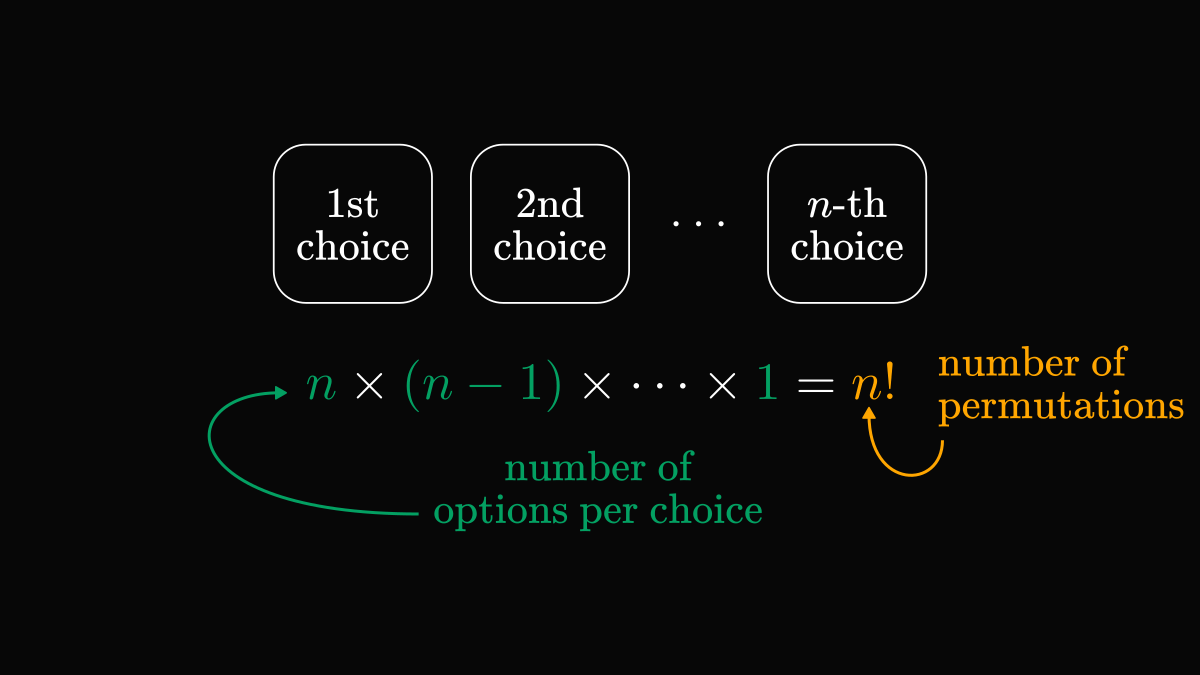# Counting permutations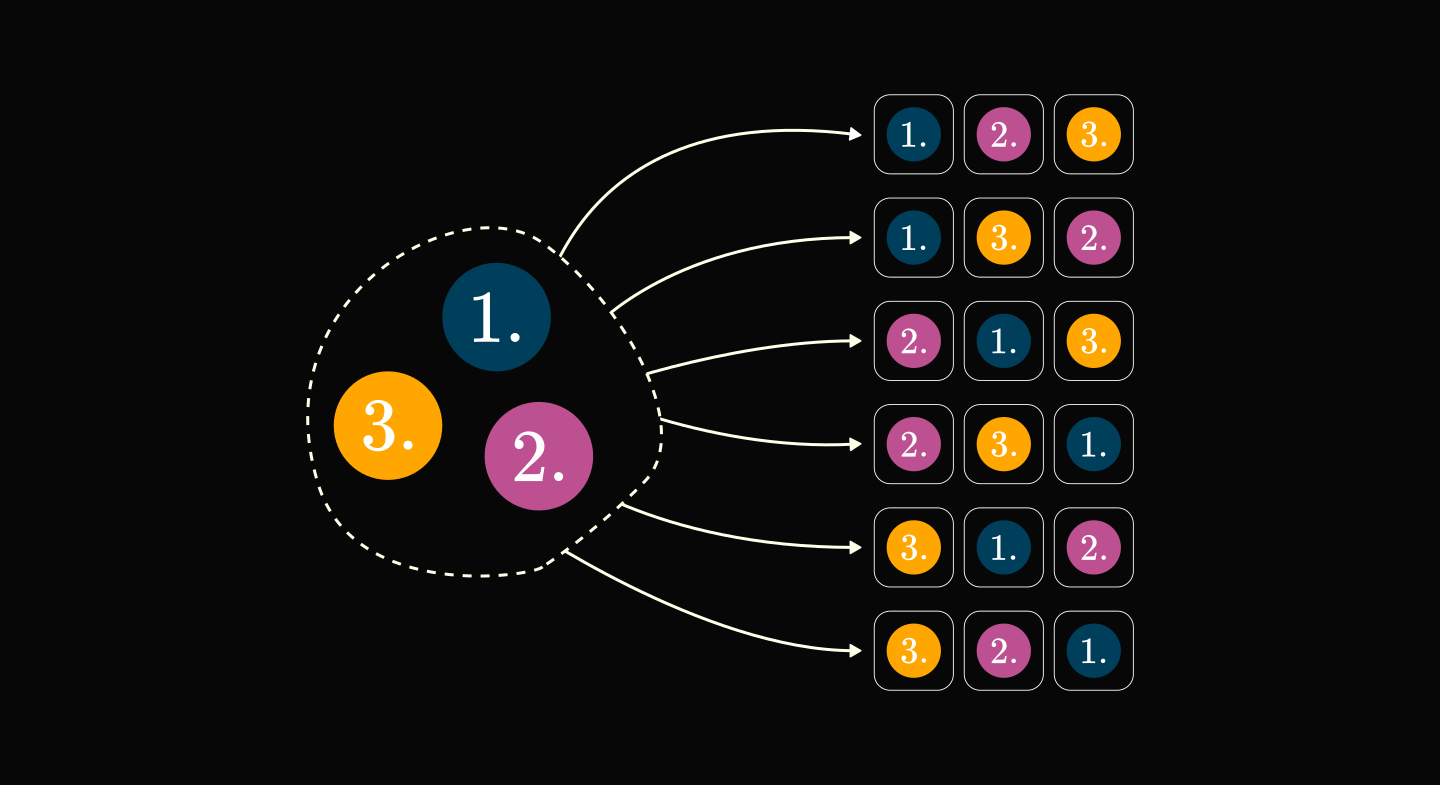Permutations are one of the fundamental building blocks of mathematics, used everywhere from algebra through probability to computer science. Knowing how to count them is an essential skill for everyone, teaching us a useful thinking pattern.

This is how to do it, step by step.

For simplicity, let's study a special case: how many ways are there to order three numbered balls?

One such ordering is called a permutation. We are essentially counting the permutations of a set with three elements.The idea is the following: when picking the first element, we have three options: 1, 2, and 3. After our first pick, say 3, we have one option less: 1 and 2. After our second pick, say 2, the third one is given since we only have one option left: 1.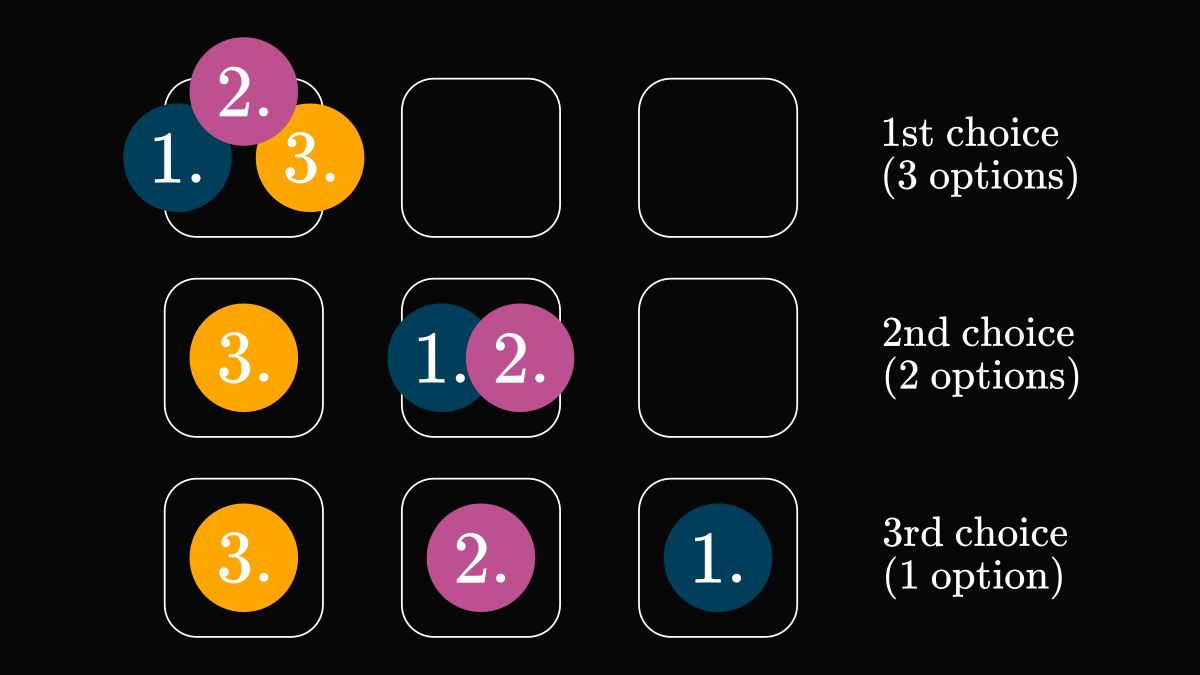This process can be visualized by a tree graph, where the levels represent choices. How many leaves are there in total?

The root has three branches, each corresponding to our 1st choice. Going one step further, each node has two branches, corresponding to the 2nd choice.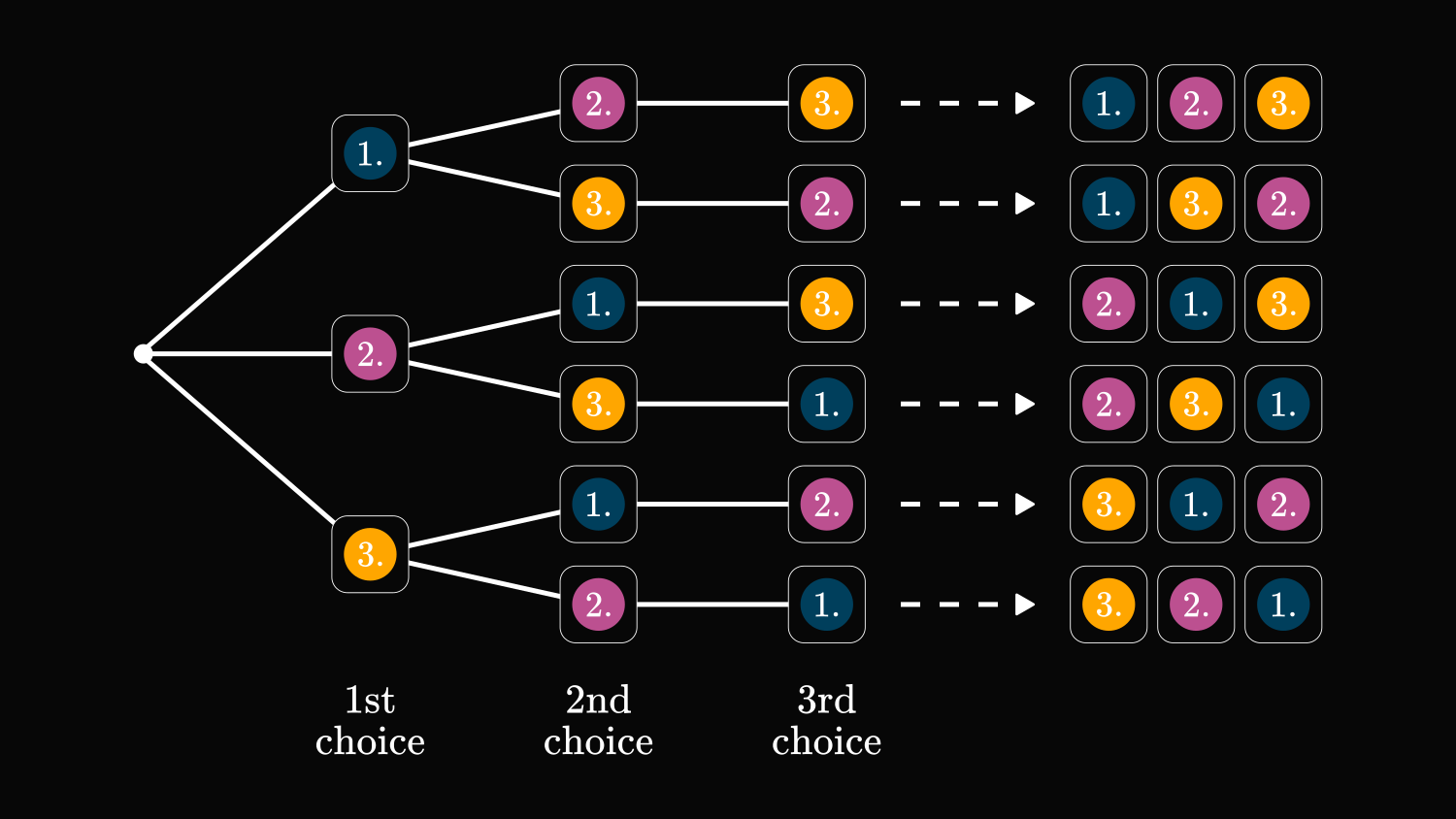To calculate the number of leaves, we have to multiply together the number of branches for a node per level. Thus, there are 3 · 2 · 1 = 6 permutations in total.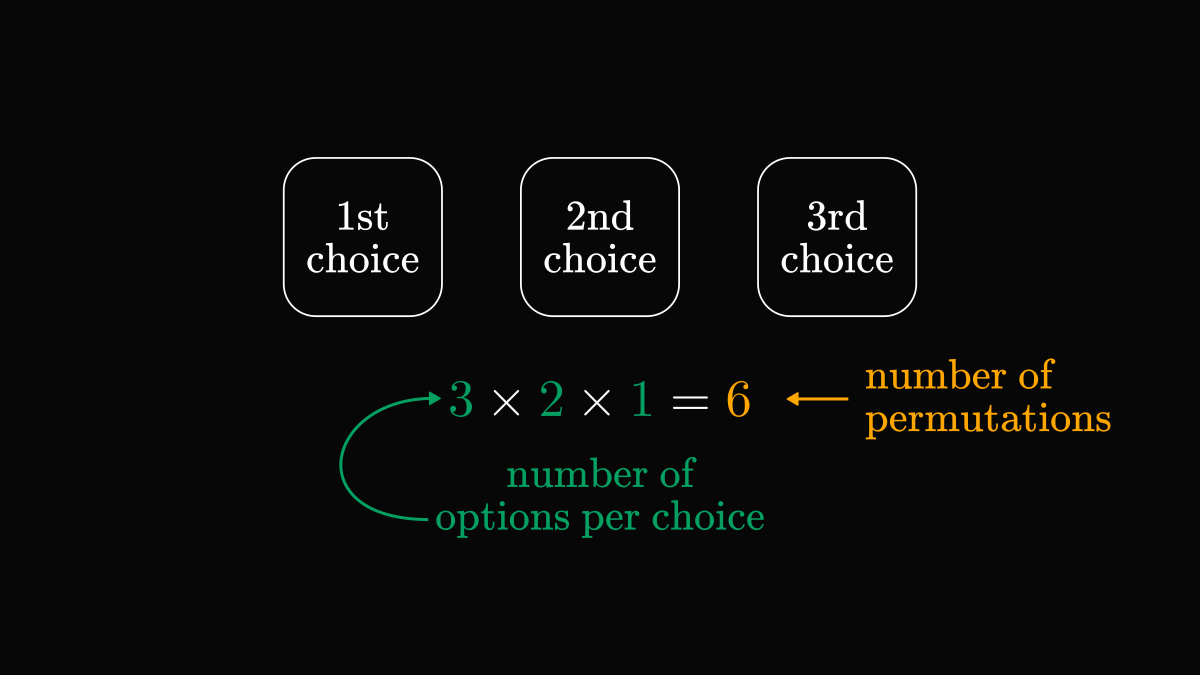The general case is the same. If we want to order n elements, we have n! possible ways, obtained by multiplying together our number of options per choice.